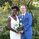4811瀏覽
The Ultimate Moving Average was created by myself and @RedKTrader and I can proudly say that this is the holy grail of moving averages. Not only does this moving average react to the current price trends like a normal moving average but we have also included the ability to react to volume , momentum, and volatility . The only thing this moving average can't do is wash your car.

The Ultimate Moving Average doesn't even use a set length so it is fully adaptable to any type of market whether it is choppy or trending. It tightens during volatile markets and loosens during choppy markets. I have included 3 of the main moving averages of a fixed length of 20 days to show you just how much better our moving average is.

The overall concept of this moving average was to fully adapt to any and all changes of the underlying stock. We used my Variable Power Weighted Moving Average as a base and changed the script to adapt to momentum instead. The idea behind this was when momentum reaches an extreme in either direction we tighten the moving average to be able to react accordingly. We then used the idea behind my Variable Length Moving Average to be able to react to volatility and make the length itself into a separate variable.

All of this work combined to create the most reactive moving average out there and I guarantee you will be using this in your daily trading! Let me know if there are any other scripts you would like to see me publish.

## 評論greenroomtimes
@greenroomtimes, if you shorten the distance between the min and max length then it lags more and more so really up to you. As far as the optimal time range and interval I designed this to work perfectly with any time range or interval and so far I haven't found a timeframe that doesn't work well with this indicatorI converted this into a function for integrating into other indicators. It seems to work, but feel free to point out any errors.

uma(src_, acc_, maxLength_, minLength_, smoothLength_) =>
mean = 0.0, stdDev = 0.0
mean := sma(src_, maxLength_)
stdDev := stdev(src_, maxLength_)
a = 0.0, b = 0.0, c = 0.0, d = 0.0
a := mean - (1.75 * stdDev)
b := mean - (0.25 * stdDev)
c := mean + (0.25 * stdDev)
d := mean + (1.75 * stdDev)

length = 0
length := src_ >= b and src_ <= c ? nz(length, maxLength_) + 1 : src_ < a or src_ > d ? nz(length, maxLength_) - 1 : nz(length, maxLength_)
length := max(min(length, maxLength_), minLength_)
len = 0
len := round(length)

// We are providing a dynamic weight depending on the current momentum
// When momentum is at an extreme for overbought/oversold then we give more weight to the current price
mf = 0.0, mfScaled = 0.0, p = 0.0
mf := na(volume) or volume == 0 ? rsi(src_, len) : mfi(src_, len)
mfScaled := (mf * 2) - 100
p := acc_ + (abs(mfScaled) / 25)

sum = 0.0, weightSum = 0.0
for i = 0 to len - 1
weight = pow(len - i, p)
sum := sum + (src_ * weight)
weightSum := weightSum + weight
uma = wma(sum / weightSum, smoothLength_)AtomOfScent
@AtomOfScent, looks great and I'm not seeing any obvious errorsThis looks great! Nice to see the collaboration.
The only feedback I can think of is that the accelerator seems to work with values less than 1 and the step size seems overly small so a min value 0.1 and step of 0.25-0.5 might be better.AtomOfScent
@AtomOfScent, Thanks! The accelerator part was actually the work of @RedKTrader so I will let him respond to those suggestions